## Homework Problems

1. An electron is bound in a harmonic oscillator potential. Small electric fields in thedirection are applied to the system. Find the lowest order nonzero shifts in the energies of the ground state and the first excited state if a constant fieldis applied. Find the same shifts if a fieldis applied.

2. A particle is in a box from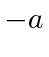to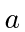in one dimension. A small additional potential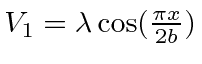is applied. Calculate the energies of the first and second excited states in this new potential.

3. The proton in the hydrogen nucleus is not really a point particle like the electron is. It has a complicated structure, but, a good approximation to its charge distribution is a uniform charge density over a sphere of radius 0.5 fermis. Calculate the effect of this potential change for the energy of the ground state of hydrogen. Calculate the effect for thestates.

4. Consider a two dimensional harmonic oscillator problem described by the Hamiltonian. Calculate the energy shifts of the ground state and the degenerate first excited states, to first order, if the additional potential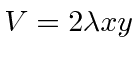is applied. Now solve the problem exactly. Compare the exact result for the ground state to that from second order perturbation theory.

5. Prove thatby starting from the expectation value of the commutatorin the stateand summing over all energy eigenstates. Assume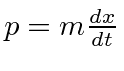and write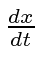in terms of the commutatorto get the result.

6. If the general form of the spin-orbit coupling for a particle of massand spin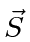moving in a potentialis, what is the effect of that coupling on the spectrum of a three dimensional harmonic oscillator? Compute the relativistic correction for the ground state of the three dimensional harmonic oscillator.

Jim Branson 2013-04-22